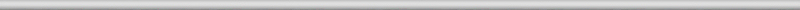## May 12, 2008

### Pattern recognition in computer vision, part 2

Let’s review part 1 first. If you have a 100×100 gray scale image, it is simply a table of 100×100 = 10,000 numbers. You rearrange the rows of this table into a 10,000-vector and represent the image as a point in the 10,000-dimensional Euclidean space. This enables you to measure distances between images, discover patterns, match images, etc. Now, what is wrong with this approach?

Suppose A, B, and C are images with a single black pixel in the left upper corner, next to it, and the right bottom corner respectively. Then, the distances will be the equal: d(A,B) = d(B,C) = d(C,A), no matter how you define the distance d(,) between points in this space. The conclusion: if A and B are in the same cluster, then so is C. So adjacency of pixels and distance between them is lost in this representation!

Of course this can be explained, as follows. The three images are essentially blank so it’s not surprising that they are close to the blank image and to each other. So as long as pixels are “small” the difference between these four images is justifiably negligible.

Of course, “small” pixels means “small” with respect to the size of the image. This means high resolution. High resolution means larger image (for the same “physical” object), which means higher dimension of the Euclidean space, which means higher computational costs. Not a good sign.

To take this line of thought all the way to the end, we have to ask the question: what if we keep increasing resolution?

The image will simply turn into an exact copy of the “physical” object. Initially, the image is a table of numbers. Now, you can think of the table as a rectangle subdivided into small squares, then the image is a function to the reals constant on each of these squares. As the resolution grows, the rectangle remains the same but the squares become smaller. In the end we have a - possibly continuous – function (as the limit of this sequence of functions). This is the “real” image and the rest are its approximations.

It’s not as clear what happens to the representations of images in the Euclidean space. The dimension of this space grows and in the end becomes infinite! It also seems that this new space should be made of infinite strings of numbers. That does not work out.

Indeed, consider this (“real”) image: a white square with a black upper left quarter. Let’s represent it first as a 2×2 image. Then in the 4-dimensional Euclidean space this image is (1,0,0,0). Now let’s increase the resolution. If this is a 4×4 image, it is (1,1,0,0,1,1,0,0,..,0) in the 16-dimensional space. In the 32-dimensional space it’s (1,1,1,1,0,0,0,0,1,1,1,1,0,0,0,0,1,1,1,1,0,…,0). You can see the pattern. But what is the end result (as the limit of this sequence of points)? It can’t be (1,1,1,…), can it? It definitely isn’t the original image. That image can’t even be represented as a string of numbers, not in any obvious way…

OK, these are just signs that there may be something wrong with this approach. A more tangible problem is that unless the two images are aligned first, there is no way to use this representation to discover that they depict the same or similar thing. About that in the next post.